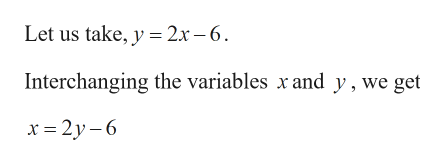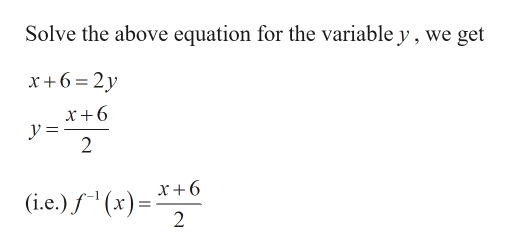Question
8 views

Find

f −1(x).
f(x) = 2x − 6
f −1(x)=
check_circle

Step 1

Given:

Step 2

Calculation:

To find the inverse of the given function:help_outlineImage TranscriptioncloseLet us take, y = 2x – 6. Interchanging the variables x and y, we get x = 2y – 6 fullscreen
Step 3help_outlineImage TranscriptioncloseSolve the above equation for the variable y , we get x+6=2y x+6 y = x+6 (i.e.) f " (x)=- fullscreen

### Want to see the full answer?

See Solution

#### Want to see this answer and more?

Solutions are written by subject experts who are available 24/7. Questions are typically answered within 1 hour.*

See Solution
*Response times may vary by subject and question.
Tagged in
MathAlgebra

### Other